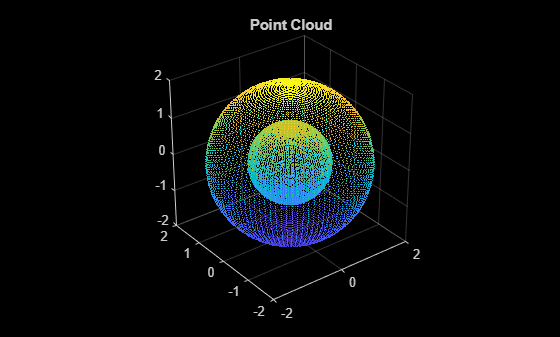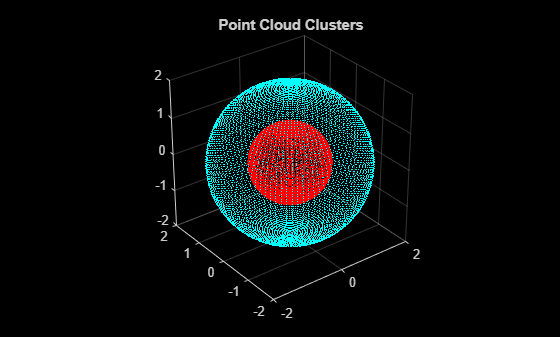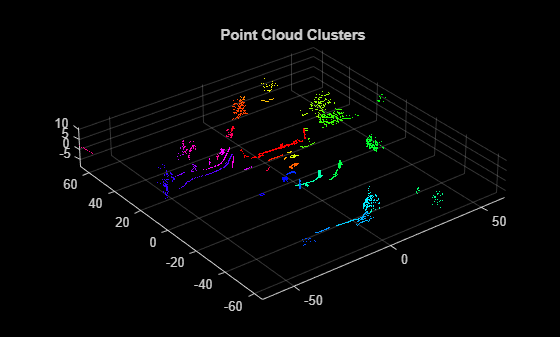# pcsegdist

Segment point cloud into clusters based on Euclidean distance

## Syntax

``labels = pcsegdist(ptCloud,minDistance)``
``[labels,numClusters] = pcsegdist(ptCloud,minDistance)``
``[___] = pcsegdist(___,Name=Value)``

## Description

````labels = pcsegdist(ptCloud,minDistance)` segments a point cloud into clusters, with a minimum Euclidean distance of `minDistance` between points from different clusters. `pcsegdist` assigns an integer cluster label to each point in the point cloud, and returns the `labels` of all points.```

example

````[labels,numClusters] = pcsegdist(ptCloud,minDistance)` also returns the number of clusters.```
````[___] = pcsegdist(___,Name=Value)` sets properties using name-value arguments. For example, ```labels = pcsegdist(ptCloud,minDistance,NumClusterPoints=[1,Inf])``` sets the minimum and maximum number of points in each cluster to `[1,Inf]`.```

## Examples

collapse all

Create two concentric spheres and combine them.

```[X,Y,Z] = sphere(100); loc1 = [X(:),Y(:),Z(:)]; loc2 = 2*loc1; ptCloud = pointCloud([loc1;loc2]); pcshow(ptCloud) title('Point Cloud')```Set the minimum Euclidean distance between clusters.

`minDistance = 0.5;`

Segment the point cloud.

`[labels,numClusters] = pcsegdist(ptCloud,minDistance);`

Plot the labeled results. The points are grouped into two clusters.

```pcshow(ptCloud.Location,labels) colormap(hsv(numClusters)) title('Point Cloud Clusters')```Load an organized lidar point cloud in the workspace.

`ld = load('drivingLidarPoints.mat');`

Detect the ground plane. Distance is measured in meters.

```maxDistance = 0.9; referenceVector = [0 0 1]; [~,inliers,outliers] = pcfitplane(ld.ptCloud,maxDistance,referenceVector);```

Remove the ground plane points.

`ptCloudWithoutGround = select(ld.ptCloud,outliers);`

Cluster the point cloud with a minimum of 10 points per cluster.

```minDistance = 2; minPoints = 10; [labels,numClusters] = pcsegdist(ptCloudWithoutGround,minDistance,'NumClusterPoints',minPoints);```

Remove the points with a label value of `0`.

```idxValidPoints = find(labels); labelColorIndex = labels(idxValidPoints); segmentedPtCloud = select(ptCloudWithoutGround,idxValidPoints);```

Plot the labeled results.

```figure colormap(hsv(numClusters)) pcshow(segmentedPtCloud.Location,labelColorIndex) title('Point Cloud Clusters')```## Input Arguments

collapse all

Point cloud, specified as a `pointCloud` object.

Minimum Euclidean distance between points from two different clusters, specified as a positive scalar.

Data Types: `single` | `double`

### Name-Value Arguments

Example: `ParallelNeighborSearch`=`false` sets the `ParallelNeighborSearch` to `false`.

Specify optional pairs of arguments as `Name1=Value1,...,NameN=ValueN`, where `Name` is the argument name and `Value` is the corresponding value. Name-value arguments must appear after other arguments, but the order of the pairs does not matter.

Minimum and maximum number of points in each cluster, specified as a scalar or a 2-element vector of the form [minPoints,maxPoints]. When you specify `NumClusterPoints` as a scalar, the maximum number of points in the cluster is unrestricted. The function sets `labels` to `0` when clusters are outside of the specified range.

Parallel neighbor search to segment point cloud data, specified as `true` or `false`. Set this property to `true` when you expect there to be approximately 50 clusters or more with fewer than 100 points per cluster.

A parallel neighbor search can improve segmentation speed for some datasets. Improved speed depends on the dataset and the value of the `minDistance` input.

## Output Arguments

collapse all

Cluster labels, returned as one of the following.

• If the point cloud, `ptCloud`, stores point locations as an unorganized M-by-3 matrix, then `labels` is an M-by-1 vector.

• If the point cloud, `ptCloud`, stores point locations as an organized M-by-N-by-3 array, then `labels` is an M-by-N matrix.

Each point in the point cloud has a cluster label, specified by the corresponding element in `labels`. The value of each label is an integer from `0` to the number of clusters of valid points, `numClusters`. The value `0` is reserved for invalid points, such as points with `Inf` or `NaN` coordinates.

Number of clusters, returned as a positive integer. The number of clusters does not include the cluster corresponding to invalid points and excludes the label value, `0`, which is reserved for invalid points.

## Version History

Introduced in R2018a